# ATM 623: Climate Modeling¶

Brian E. J. Rose, University at Albany

# Lecture 11: Insolation¶

This document uses the interactive IPython notebook format (now also called Jupyter). The notes can be accessed in several different ways:

Many of these notes make use of the climlab package, available at https://github.com/brian-rose/climlab

## 1. Distribution of insolation¶

These notes closely follow section 2.7 of Dennis L. Hartmann, "Global Physical Climatology", Academic Press 1994.

The amount of solar radiation incident on the top of the atmosphere (what we call the "insolation") depends on

• latitude
• season
• time of day

This insolation is the primary driver of the climate system. Here we will examine the geometric factors that determine insolation, focussing primarily on the daily average values.

### Solar zenith angle¶

We define the solar zenith angle $\theta_s$ as the angle between the local normal to Earth's surface and a line between a point on Earth's surface and the sun.

In :
from IPython.display import Image
Image('../images/Hartmann_Fig2.5.png')

Out: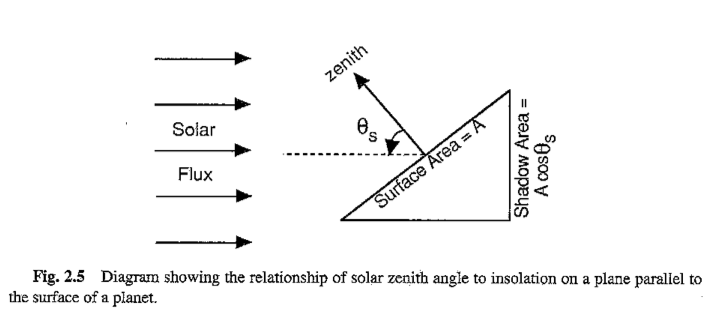From the above figure (reproduced from Hartmann's book), the ratio of the shadow area to the surface area is equal to the cosine of the solar zenith angle.

### Instantaneous solar flux¶

We can write the solar flux per unit surface area as

$$Q = S_0 \left( \frac{\overline{d}}{d} \right)^2 \cos \theta_s$$

where $\overline{d}$ is the mean distance for which the flux density $S_0$ (i.e. the solar constant) is measured, and $d$ is the actual distance from the sun.

Question:

• what factors determine $\left( \frac{\overline{d}}{d} \right)^2$ ?
• under what circumstances would this ratio always equal 1?

### Calculating the zenith angle¶

Just like the flux itself, the solar zenith angle depends latitude, season, and time of day.

#### Declination angle¶

The seasonal dependence can be expressed in terms of the declination angle of the sun: the latitude of the point on the surface of Earth directly under the sun at noon (denoted by $\delta$).

$\delta$ currenly varies between +23.45º at northern summer solstice (June 21) to -23.45º at northern winter solstice (Dec. 21).

#### Hour angle¶

The hour angle $h$ is defined as the longitude of the subsolar point relative to its position at noon.

#### Formula for zenith angle¶

With these definitions and some spherical geometry (see Appendix A of Hartmann's book), we can express the solar zenith angle for any latitude $\phi$, season, and time of day as

$$\cos \theta_s = \sin \phi \sin \delta + \cos\phi \cos\delta \cos h$$

#### Sunrise and sunset¶

If $\cos\theta_s < 0$ then the sun is below the horizon and the insolation is zero (i.e. it's night time!)

Sunrise and sunset occur when the solar zenith angle is 90º and thus $\cos\theta_s=0$. The above formula then gives

$$\cos h_0 = - \tan\phi \tan\delta$$

where $h_0$ is the hour angle at sunrise and sunset.

#### Polar night¶

Near the poles special conditions prevail. Latitudes poleward of 90º-$\delta$ are constantly illuminated in summer, when $\phi$ and $\delta$ are of the same sign. Right at the pole there is 6 months of perpetual daylight in which the sun moves around the compass at a constant angle $\delta$ above the horizon.

In the winter, $\phi$ and $\delta$ are of opposite sign, and latitudes poleward of 90º-$|\delta|$ are in perpetual darkness. At the poles, six months of daylight alternate with six months of daylight.

At the equator day and night are both 12 hours long throughout the year.

### Daily average insolation¶

Substituting the expression for solar zenith angle into the insolation formula gives the instantaneous insolation as a function of latitude, season, and time of day:

$$Q = S_0 \left( \frac{\overline{d}}{d} \right)^2 \Big( \sin \phi \sin \delta + \cos\phi \cos\delta \cos h \Big)$$

which is valid only during daylight hours, $|h| < h_0$, and $Q=0$ otherwise (night).

To get the daily average insolation, we integrate this expression between sunrise and sunset and divide by 24 hours (or $2\pi$ radians since we express the time of day in terms of hour angle):

$$\overline{Q}^{day} = \frac{1}{2\pi} \int_{-h_0}^{h_0} Q ~dh$$$$= \frac{S_0}{2\pi} \left( \frac{\overline{d}}{d} \right)^2 \int_{-h_0}^{h_0} \Big( \sin \phi \sin \delta + \cos\phi \cos\delta \cos h \Big) ~ dh$$

which is easily integrated to get our formula for daily average insolation:

$$\overline{Q}^{day} = \frac{S_0}{\pi} \left( \frac{\overline{d}}{d} \right)^2 \Big( h_0 \sin\phi \sin\delta + \cos\phi \cos\delta \sin h_0 \Big)$$

where the hour angle at sunrise/sunset $h_0$ must be in radians.

### The daily average zenith angle¶

It turns out that, due to optical properties of the Earth's surface (particularly bodies of water), the surface albedo depends on the solar zenith angle. It is therefore useful to consider the average solar zenith angle during daylight hours as a function of latidude and season.

The appropriate daily average here is weighted with respect to the insolation, rather than weighted by time. The formula is

$$\overline{\cos\theta_s}^{day} = \frac{\int_{-h_0}^{h_0} Q \cos\theta_s~dh}{\int_{-h_0}^{h_0} Q ~dh}$$
In :
Image('../images/Hartmann_Fig2.8.png')

Out: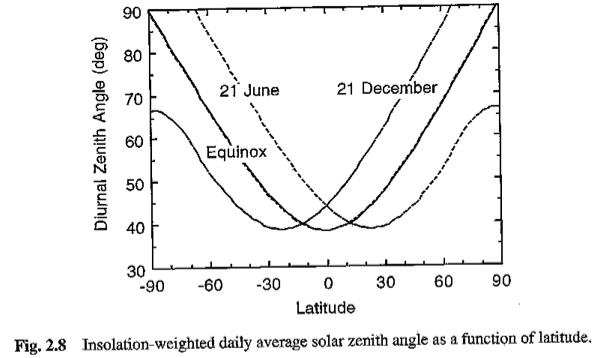The average zenith angle is much higher at the poles than in the tropics. This contributes to the very high surface albedos observed at high latitudes.

## 2. Computing daily insolation with climlab¶

Here are some examples calculating daily average insolation at different locations and times.

These all use a function called daily_insolation in the module climlab.solar.insolation.py to do the calculation. The code implements the above formulas to calculates daily average insolation anywhere on Earth at any time of year.

The code takes account of orbital parameters to calculate current Sun-Earth distance.

To look at past orbital variations and their effects on insolation, we use the module climlab.solar.orbital.py which accesses tables of values for the past 5 million years. We can easily lookup parameters for any point in the past and pass these to daily_insolation.

In :
%matplotlib inline
import numpy as np
import matplotlib.pyplot as plt
import netCDF4 as nc
from climlab import constants as const
from climlab.solar.insolation import daily_insolation
from climlab.solar.orbital import OrbitalTable


First, get a little help on using the daily_insolation function:

In :
help(daily_insolation)

Help on function daily_insolation in module climlab.solar.insolation:

daily_insolation(lat, day, orb={'ecc': 0.017236, 'long_peri': 281.37, 'obliquity': 23.446}, S0=None, day_type=1)
Compute daily average insolation given latitude, time of year and orbital parameters.

Orbital parameters can be computed for any time in the last 5 Myears with
ecc,long_peri,obliquity = orbital.lookup_parameters(kyears)

Inputs:
lat:      Latitude in degrees (-90 to 90).
day:      Indicator of time of year, by default day 1 is Jan 1.
orb:    a dictionary with three members (as provided by orbital.py)
ecc:      eccentricity (dimensionless)
long_peri:    longitude of perihelion (precession angle) (degrees)
obliquity:  obliquity angle (degrees)
S0:       Solar constant in W/m^2, will try to read from constants.py
day_type: Convention for specifying time of year (+/- 1,2) [optional].
day_type=1 (default): day input is calendar day (1-365.24), where day 1
is January first.  The calendar is referenced to the vernal equinox
which always occurs at day 80.
day_type=2: day input is solar longitude (0-360 degrees). Solar
longitude is the angle of the Earth's orbit measured from spring
equinox (21 March). Note that calendar days and solar longitude are
not linearly related because, by Kepler's Second Law, Earth's
angular velocity varies according to its distance from the sun.
Default values for orbital parameters are present-day

Output:
Fsw = Daily average solar radiation in W/m^2.

Dimensions of output are (lat.size, day.size, ecc.size)

Code is fully vectorized to handle array input for all arguments.
Orbital arguments should all have the same sizes.
This is automatic if computed from orbital.OrbitalTable.lookup_parameters()

e.g. to compute the timeseries of insolation at 65N at summer solstice over the past 5 Myears
from climlab.orbital import OrbitalTable
table = OrbitalTable()
years = np.linspace(0, 5000, 5001)
orb = table.lookup_parameters( years )
S65 = orbital.daily_insolation( 65, 172, orb )



Here are a few simple examples.

First, compute the daily average insolation at 45ºN on January 1:

In :
daily_insolation(45,1)

Out:
array(123.9532155180746)

Same location, July 1:

In :
daily_insolation(45,181)

Out:
array(482.356497522712)

We could give an array of values. Let's calculate and plot insolation at all latitudes on the spring equinox = March 21 = Day 80

In :
lat = np.linspace(-90., 90., 30.)
Q = daily_insolation(lat, 80)
plt.plot(lat,Q)
plt.xlim(-90,90)
plt.xticks([-90,-60,-30,-0,30,60,90])
plt.xlabel('Latitude')
plt.grid()
plt.title('Daily average insolation on March 21')

Out:
<matplotlib.text.Text at 0x111eca890>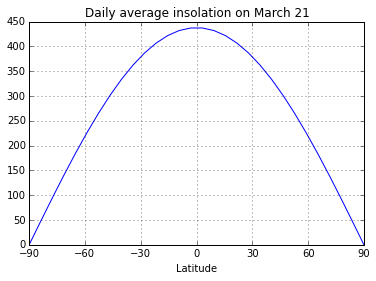### In-class exercises¶

Try to answer the following questions before reading the rest of these notes.

• What is the daily insolation today here at Albany (latitude 42.65ºN)?
• What is the annual mean insolation at the latitude of Albany?
• At what latitude and a what time of year does the maximum insolation occur?
• What latitude is experiencing either polar sunrise or polar sunset today?

### Global, seasonal distribution of insolation (present-day orbital parameters)¶

Calculate an array of insolation over the year and all latitudes (for present-day orbital parameters). We'll use a dense grid in order to make a nice contour plot

In :
lat = np.linspace( -90., 90., 500. )
days = np.linspace(0, const.days_per_year, 365. )
Q = daily_insolation( lat, days )


And make a contour plot of Q as function of latitude and time of year.

In :
ax = plt.figure( figsize=(10,8) ).add_subplot(111)
CS = ax.contour( days, lat, Q , levels = np.arange(0., 600., 50.) )
ax.clabel(CS, CS.levels, inline=True, fmt='%r', fontsize=10)
ax.set_xlabel('Days since January 1', fontsize=16 )
ax.set_ylabel('Latitude', fontsize=16 )
ax.set_title('Daily average insolation', fontsize=24 )
ax.contourf ( days, lat, Q, levels=[0., 0.] )
plt.show()

/Users/Brian/anaconda/lib/python2.7/site-packages/matplotlib/text.py:52: UnicodeWarning: Unicode equal comparison failed to convert both arguments to Unicode - interpreting them as being unequal
if rotation in ('horizontal', None):
/Users/Brian/anaconda/lib/python2.7/site-packages/matplotlib/text.py:54: UnicodeWarning: Unicode equal comparison failed to convert both arguments to Unicode - interpreting them as being unequal
elif rotation == 'vertical':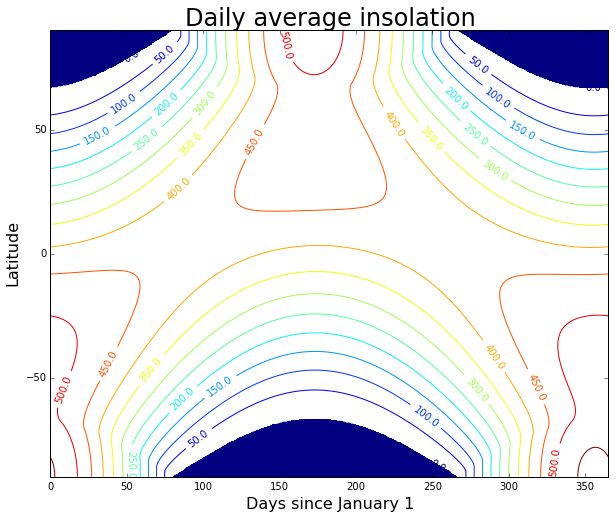### Time and space averages¶

Take the area-weighted global, annual average of Q...

In :
Qaverage = np.average(np.mean(Q, axis=1), weights=np.cos(np.deg2rad(lat)))
print 'The annual, global average insolation is %.2f W/m2.' %Qaverage

The annual, global average insolation is 341.38 W/m2.


Also plot the zonally averaged insolation at a few different times of the year:

In :
summer_solstice = 170
winter_solstice = 353
ax.plot( lat, Q[:,(summer_solstice, winter_solstice)] );
ax.plot( lat, np.mean(Q, axis=1), linewidth=2 )
ax.set_xbound(-90, 90)
ax.set_xticks( range(-90,100,30) )
ax.set_xlabel('Latitude', fontsize=16 );
ax.set_ylabel('Insolation (W m$^{-2}$)', fontsize=16 );
ax.grid()
plt.show()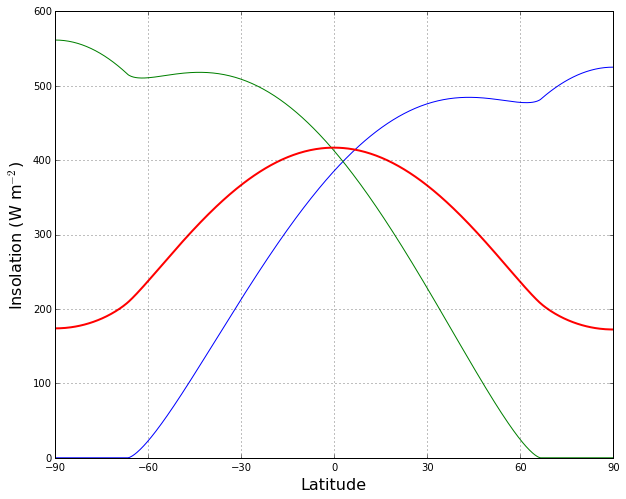[Back to ATM 623 notebook home](../index.html)

## Credits¶

The author of this notebook is Brian E. J. Rose, University at Albany.

It was developed in support of ATM 623: Climate Modeling, a graduate-level course in the Department of Atmospheric and Envionmental Sciences, offered in Spring 2015.

## Version information¶

In :
%install_ext http://raw.github.com/jrjohansson/version_information/master/version_information.py
%version_information numpy, climlab

Installed version_information.py. To use it, type: